NCERT Solutions for Class 7 Maths Chapter 8 Comparing Quantities Ex 8.2 are part of NCERT Solutions for Class 7 Maths. Here we have given NCERT Solutions for Class 7 Maths Chapter 8 Comparing Quantities Ex 8.2.

 Board CBSE Textbook NCERT Class Class 7 Subject Maths Chapter Chapter 8 Chapter Name Comparing Quantities Exercise Ex 8.2 Number of Questions Solved 10 Category NCERT Solutions

## NCERT Solutions for Class 7 Maths Chapter 8 Comparing Quantities Ex 8.2

EXERCISE 8.2

Question 1.
Convert the given fractional numbers to per cents.

(a) $$\frac { 1 }{ 8 }$$
(b) $$\frac { 5 }{ 4 }$$
(c) $$\frac { 3 }{ 40 }$$
(d) $$\frac { 2 }{ 7 }$$

Solution: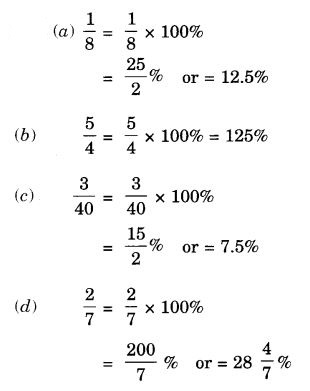Question 2.
Convert the given decimal fractions to per cents.

(a) 0.65
(b) 2.1
(c) 0.02
(d) 12.35

Solution: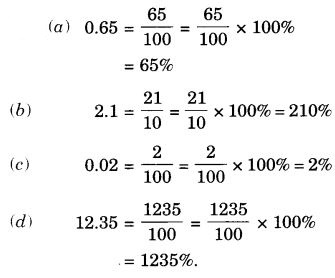Question 3.
Estimate what part of the figures is coloured and hence find the percent which is coloured.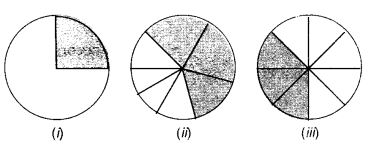Solution:Question 4.
Find:

(a) 15% of 250
(b) 1% of 1 hour
(c) 20% of ₹ 2500
(d) 75% of 1 kg.

Solution: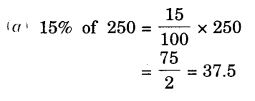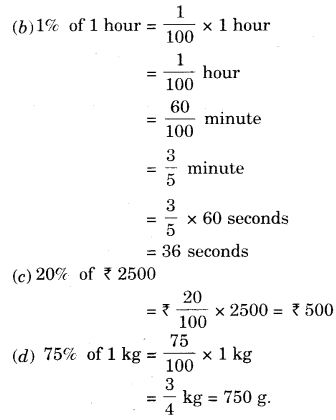Question 5.
Find the whole quantity if

(a) 5% of it is 600.
(b) 12% of it is ₹ 1080.
(c) 40% of it is 500 km.
(d) 70% of it is 14 minutes.
(e) 8% of it is 40 liters.

Solution: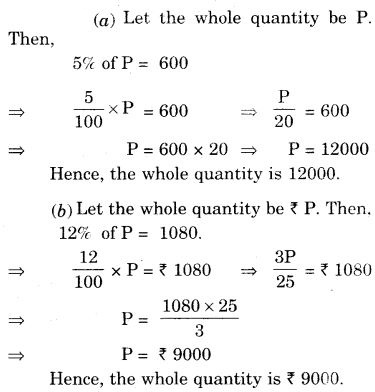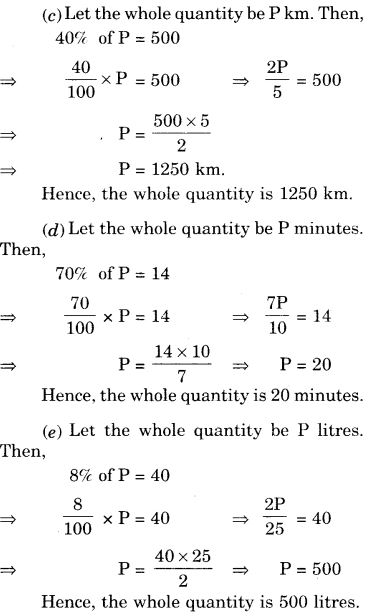Question 6.
Convert given percents to decimal fractions and also to fractions in the simplest forms.

(a) 25%
(b) 150%
(c) 20%
(d) 5%

Solution: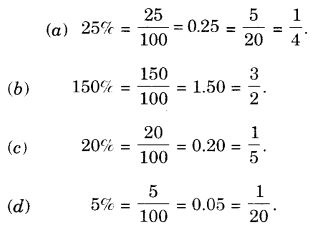Question 7.
In a city, 30% are females, 40% are males, and the remaining are children. What percent are children?
Solution:
[100% (30% + 40%)] are children
⇒ [100% – 70% ] are children
⇒ 30% are children.

Question 8.
Out of 15,000 voters in a constituency, 60% voted. Find the percentage of voters who did not vote. Can you now find how many actually did not vote?
Solution:
Percentage of voters who voted = 60%
Percentage of voters who did not vote = (100 – 60)% = 40%
Total number of voters = 15000
Number of voters who did not vote = 40% of 15000
= ( $$\frac { 40 }{ 100 }$$ × 15000 )
= 6000

Question 9.
Meeta saves ₹ 400 from her salary. If this is 10% of her salary. What is her salary?
Solution:
Let her salary be ₹ P. Then, 10% of P = 400
⇒ $$\frac { 10 }{ 100 }$$ × p = 400
⇒ $$\frac { P }{ 10 }$$ = 400
⇒ P = 400 × 10
⇒ P = 4000
Hence, her salary is ₹ 4000.

Question 10.
A local cricket team played 20 matches in one season. It won 25% of them. How many matches did they win?
Solution:
Out of 100, 25% matches are won. Then, out of 20, the number of matches that the team won
= $$\frac { 25 }{ 100 }$$ × 20
= $$\frac { 1 }{ 4 }$$ × 20
= 5

We hope the NCERT Solutions for Class 7 Maths Chapter 8 Comparing Quantities Ex 8.2 help you. If you have any query regarding NCERT Solutions for Class 7 Maths Chapter 8 Comparing Quantities Ex 8.2, drop a comment below and we will get back to you at the earliest.